Create a new printable
Answer key also includes questions
Answer key only gives the answers
No answer keyMath Worksheets
Multiplication

Sample - Click above to make a new math worksheet (PDF).
 Name _____________________________Date ___________________
Addition and Multiplication
Write the sum and then write the product.

1.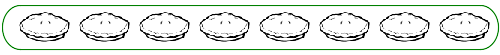8 + 8 = ______ 2 x 8 = ______
2.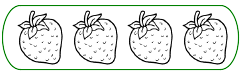4 + 4 + 4 + 4 = ______ 4 x 4 = ______
3.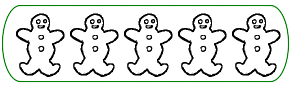5 + 5 = ______ 2 x 5 = ______
4.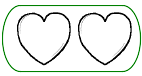2 + 2 + 2 + 2 + 2 + 2 = ______ 6 x 2 = ______
5.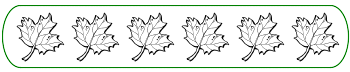6 + 6 = ______ 2 x 6 = ______
6.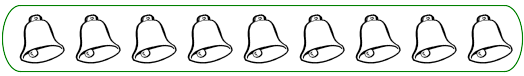9 + 9 = ______ 2 x 9 = ______
7.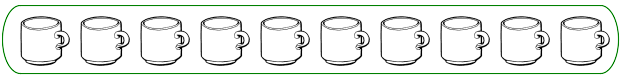10 + 10 = ______ 2 x 10 = ______
 8 * This is a pre-made sheet.Use the link at the top of the page for a printable page.
9.3 + 3 + 3 = ______ 3 x 3 = ______

 Answer Key

Sample
This is only a sample worksheet.

Create a new printable
Answer key also includes questions
Answer key only gives the answers
No answer keyMath Worksheets
Multiplication